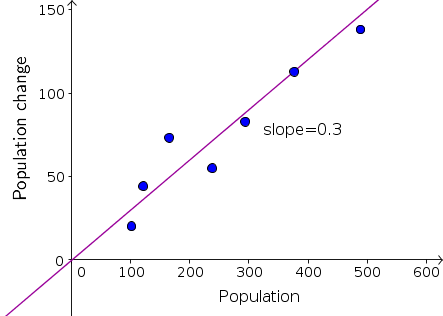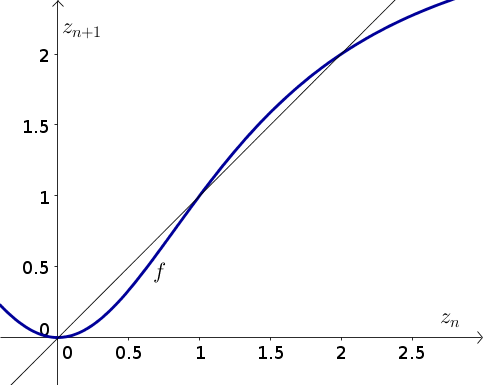# Math Insight

### Elementary discrete dynamical systems biology problems

#### Problem 1

The mass of a fish is increasing by 100 grams per year.

1. Set up a dynamical system model that describes the evolution of the fish's mass. Be sure to define your notation, including the meaning of any variable for time.
2. If the mass of a fish in year 0 is 45 grams, calculate the mass in the next 4 years. Be sure to show how you used the formula in part (a) and to write your results using the notation you defined.
3. Find all equilibria of your dynamical system.

#### Problem 2

In a population of elk, each year the number of babies is 20% of the population size at the beginning of the year. However, a disease is killing one quarter of the population each year.

1. Set up a dynamical system model that describes the evolution of the population size. Be sure to define your notation, including the meaning of any variable for time.
2. If in one year, there are 18,000 elk, calculate the number of elk for the next 4 years. (Don't worry about having fractional elk.) Be sure to show how you used the formula in part (a) and to write your results using the notation you defined.
3. Find all equilibria of your dynamical system.

#### Problem 3

The human population of the earth is currently increasing by about 1.1% per year. If it continues at this rate, how many years will it take for the population to double? If the current population is 7 billion people, in how many years will the population reach 56 billion people?

#### Problem 4

The population of Steller sea lions is decreasing at a rate of about 5% per year. If it continues at this rate, how many years will it take for the population to decline by one-half? If the current population is 40,000, in how many years will the population reach 10,000 Steller sea lions?

#### Problem 5

In the first days of life, the cells in a human embryo divide into two cells approximately every day.

1. Assuming the number of cells doubles every day, write down a dynamical system for the evolution of the number of cells. Be sure to define your notation, including the meaning of any variable for time.
2. After fertilization, the embryo consists of a single cell. Solve the dynamical system to obtain an expression for the number of cells as a function of the number of days since fertilization.
3. If a pregnancy lasts 40 weeks and the cell division continued at the same rate, how many cells would the baby have upon being born? How does this number compare to the number of atoms in the observable universe, which is estimated to be about $10^{80}$? What can you conclude about the rate of cell division during the course of the pregnancy?

#### Problem 6

1. In every 5 week period, the population size of a group of rabbits increases by a fixed fraction. Set up a dynamical system model that describes the evolution of the rabbit population. Be sure to define your notation, including the meaning of any variable for time. Your model should include an unknown parameter, which you should clearly define.
2. You count the number of rabbits in a population every 5 weeks and obtain the following data. You also calculate how much the population changed from each observation until the next.

Time (weeks)Population sizePopulation change
010120
512144
1016573
1523855
2029383
25376113
30489138
35627

You plot population change versus population size, fit the result with a line through the origin, and obtain a slope of 0.3.Based on this result, determine the unknown parameter from your model. Write down your resulting dynamical system model that describes the data. Be sure to include the initial condition equation.

3. Solve the dynamical system model to obtain an expression for the population size in any time interval. Your final result should not contain any parameter, but only the variable used for time.
4. Rewrite your solution to give the population size as a function of the number of weeks since the beginning. Based on your model, estimate the rabbit population after 35 weeks and after two years (104 weeks).

#### Problem 7

Bacteria are growing in a beaker so that the population size increases by 14.87% every minute.

1. Set up a dynamical system model that describes the evolution of the population size. Be sure to define your notation, including the meaning of any variable for time.
2. How long does it take the population size to double?
3. If the population continues to grow at this rate, by what factor does the population size increase in one hour? In two hours? In four hours?
4. An experiment is begun at midnight with just a few bacteria so that the fraction of the beaker that the bacteria occupy is approximately $0.00000005959 = 5.959 \times 10^{-8}$. With this initial condition, the bacteria completely fill the beaker after two hours, at 2 AM. At what time was the beaker half full?
5. Imagine the researchers realized before 2AM that the bacteria were about to overflow the beaker. They found three more empty beakers of the same size as the original beaker so that they had a total of four beakers to hold the bacteria. At what time did the bacteria fill all four beakers?

#### Problem 8

Without hunting, a population of deer would be growing by a factor of $a$ each year, where the parameter $a$ is a number greater than one.

1. Write down a dynamical system for the unhunted population of the deer and find all equilbiria. Are the equilibria stable or unstable?
2. Modify the dynamical system of part (a) so that $b$ deer are hunted each year, where the parameter $b$ is a positive number. Find all equilibria.
3. Modify the dynamical system of part (a) so that a fraction $c$ of the deer are hunted each year, where the parameter $c$ is a positive number. Find all equilibria. Calculate a condition on $c$ for the equilibria to be stable. Calculate a condition on $c$ for there to be more than one equilibrium.

#### Problem 9

Consider the dynamical system \begin{align*} z_{n+1}=f(z_n) \quad \text{for $n=0,1,2,3,\ldots$ ,} \end{align*} where $f$ is defined by \begin{align*} f(\bigstar)=\frac{3\bigstar^2}{2+\bigstar^2}. \end{align*} The graph of $z_{n+1}=f(z_n)$ is shown below along with the diagaonal $z_{n+1}=z_n$.1. Write down an equation for the equilibria and show that the system has the equilibria 0, 1, and 2.

2. Use cobwebbing to determine what happens to the system if the initial condition $z_0$ is slightly less than 1 and if the initial condition $z_0$ is slightly greater than 1.

3. Imagine that $z_n$ represents the firing rate in time step $n$ of a neuron in a monkey's brain. At time step zero, either a banana or a stick is shown briefly to the monkey. If the banana was shown, the neuron starts firing at a rate $z_0$ of about 1.2. If a stick was shown, the neuron starts firing at a rate $z_0$ of about 0.8.

Imagine that you don't know if the monkey was shown a stick or a banana at time step zero. However, after one of these was briefly shown to the monkey, the firing rate of the neuron has evolved for many time steps, and now the neuron is firing at a rate $z_n$ of about 2. Was the monkey shown a banana or a stick? Justify your answer using the results from the first two parts.

One you have worked on a few problems, you can compare your solutions to the ones we came up with.